# Femtoscopy of pp collisions at $\sqrt{s}=0.9$ and $7~$TeV at the LHC with two-pion Bose-Einstein correlations

We report on the high statistics two-pion correlation functions from pp collisions at $\sqrt{s}=0.9~$TeV and $\sqrt{s}=7~$TeV, measured by the ALICE experiment at the Large Hadron Collider. The correlation functions as well as the extracted source radii scale with event multiplicity and pair momentum. When analyzed in the same multiplicity and pair transverse momentum range, the correlation is similar at the two collision energies. A three-dimensional femtoscopic analysis shows an increase of the emission zone with increasing event multiplicity as well as decreasing homogeneity lengths with increasing transverse momentum. The latter trend gets more pronounced as multiplicity increases. This suggests the development of space-momentum correlations, at least for collisions producing a high multiplicity of particles. We consider these trends in the context of previous femtoscopic studies in high-energy hadron and heavy-ion collisions, and discuss possible underlying physics mechanisms. Detailed analysis of the correlation reveals an exponential shape in the outward and longitudinal directions, while the sideward remains a Gaussian. This is interpreted as a result of a significant contribution of strongly decaying resonances to the emission region shape. Significant non-femtoscopic correlations are observed, and are argued to be the consequence of "mini-jet"-like structures extending to low $p_{\rm T}$. They are well reproduced by the Monte-Carlo generators and seen also in $\pi^{+}\pi^{-}$ correlations.

Figures

## Figure 1

 Projections of the 3D Cartesian representations of the correlation functions onto the $q_{out}$, $q_{side}$ and $q_{long}$ axes for pairs with $0.2 < k_{\rm T} < 0.3~$GeV/$c$, for three multiplicity ranges. To project onto one $q$-component, the others are integrated over the range $0-0.16~$GeV/$c$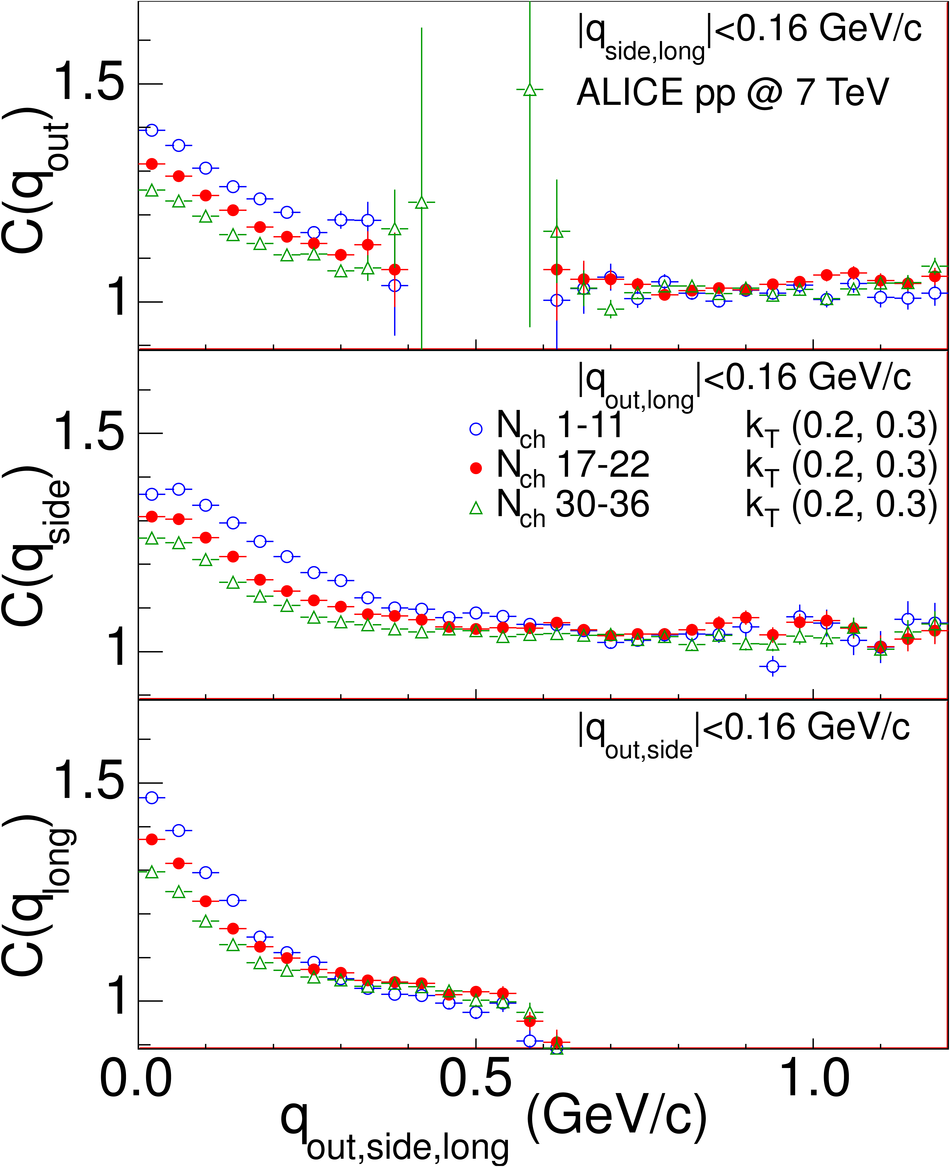## Figure 2

 Moments of the SH decomposition of the correlation functions for pairs with $0.2 < k_{\rm T} < 0.3~$GeV/$c$, for three multiplicity ranges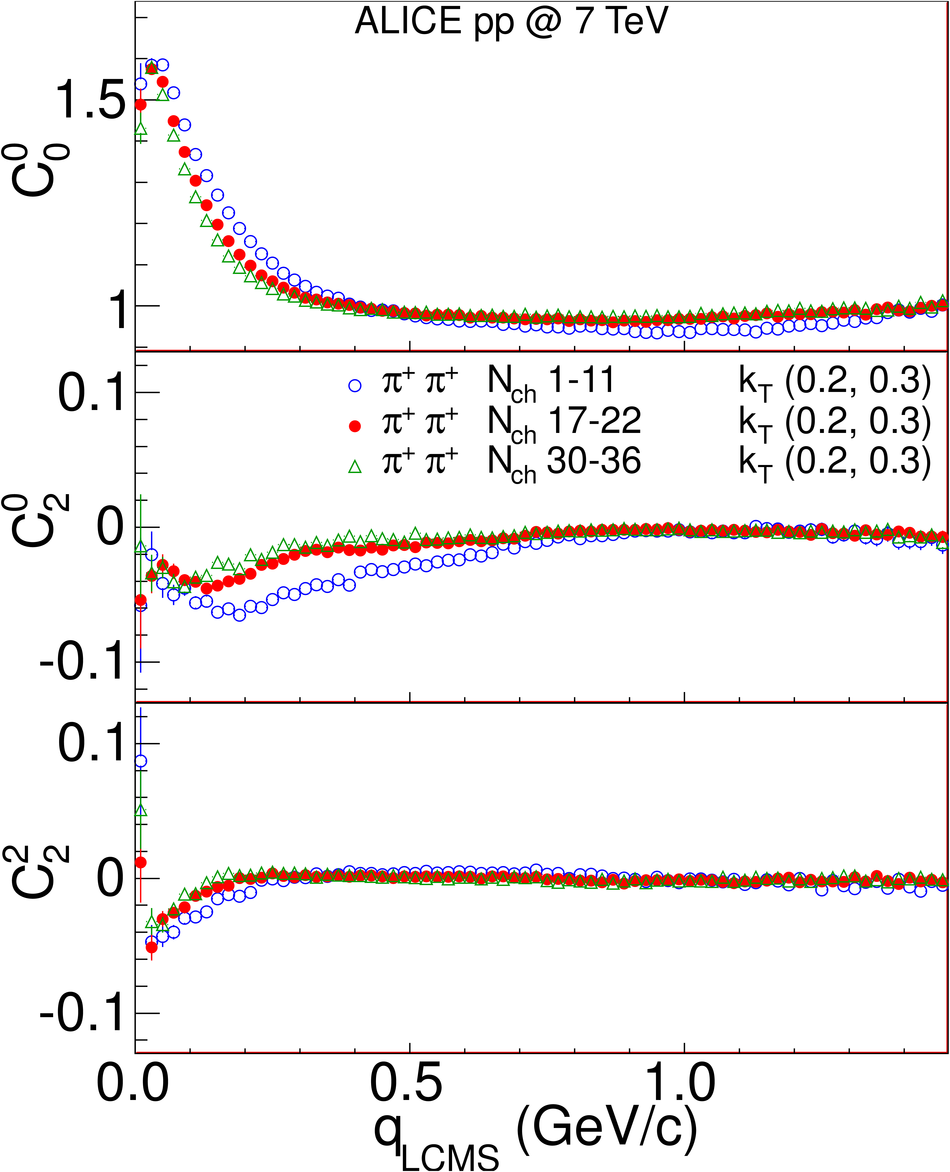## Figure 3

 Moments of the SH decomposition of the correlation functions for events with $17 \leq N_{ch} \leq 22$, for three $k_{\rm T}$ ranges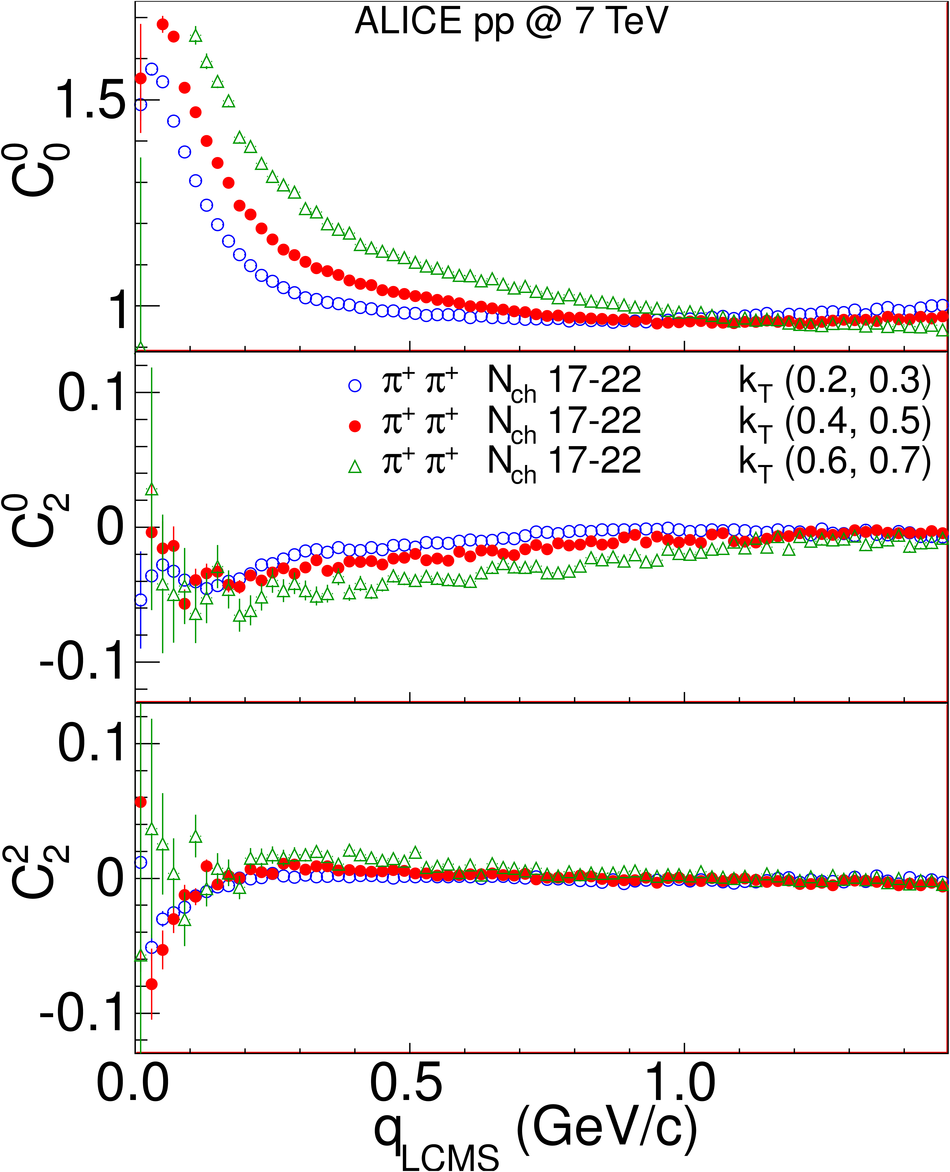## Figure 4

 Projections of the 3D Cartesian representations of the correlation functions onto the $q_{out}$, $q_{side}$ and $q_{long}$ axes, for events with $17 \leq N_{ch} \leq 22$, for three $k_{\rm T}$ ranges. To project onto one $q$-component, the others are integrated over the range $0-0.16~$GeV/$c$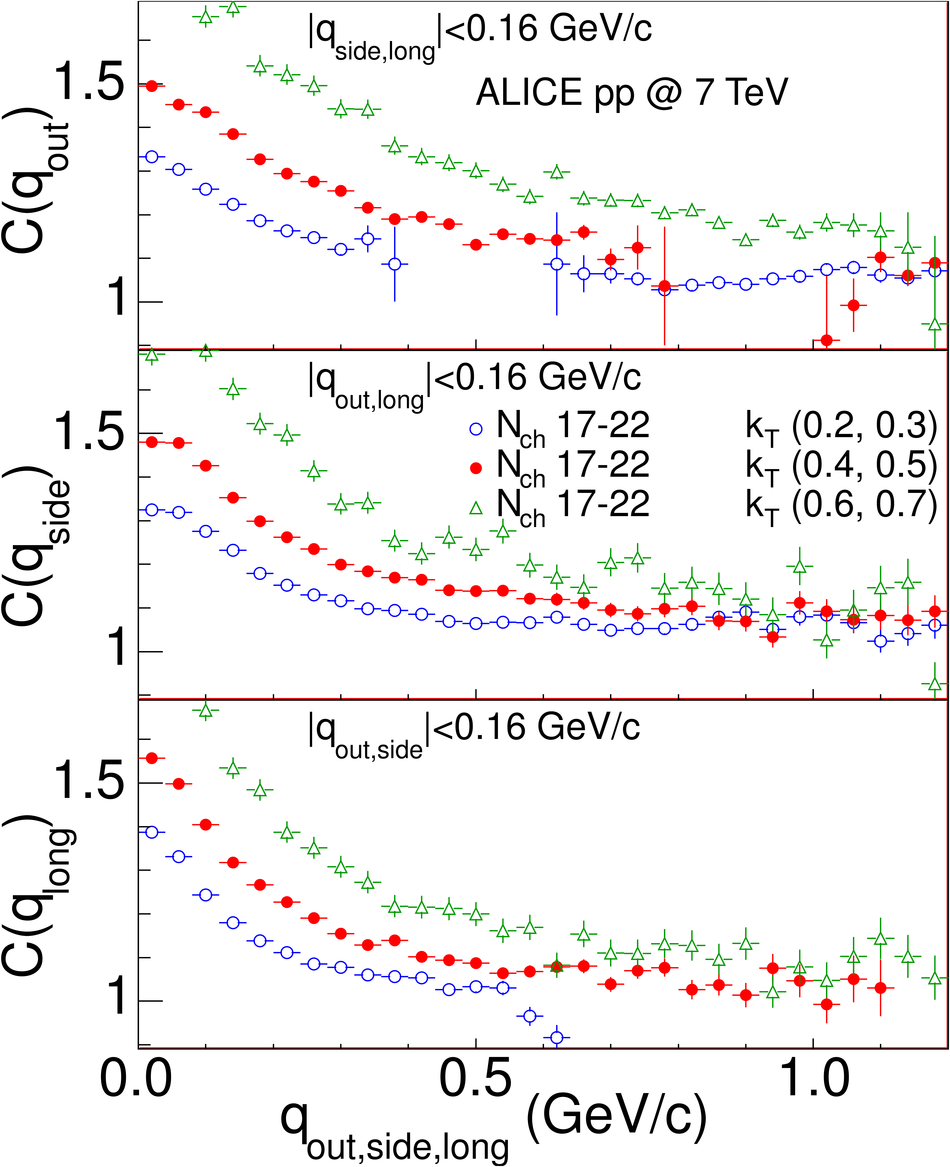## Figure 5

 Moments of the SH decomposition of the correlation functions for events with $12 \leq N_{ch} \leq 16$, pairs with $0.3 < k_{\rm T} < 0.4~$GeV/$c$. Open symbols are for $\sqrt{s} = 0.9~$TeV collisions, closed symbols for $\sqrt{s} = 7~$TeV collisions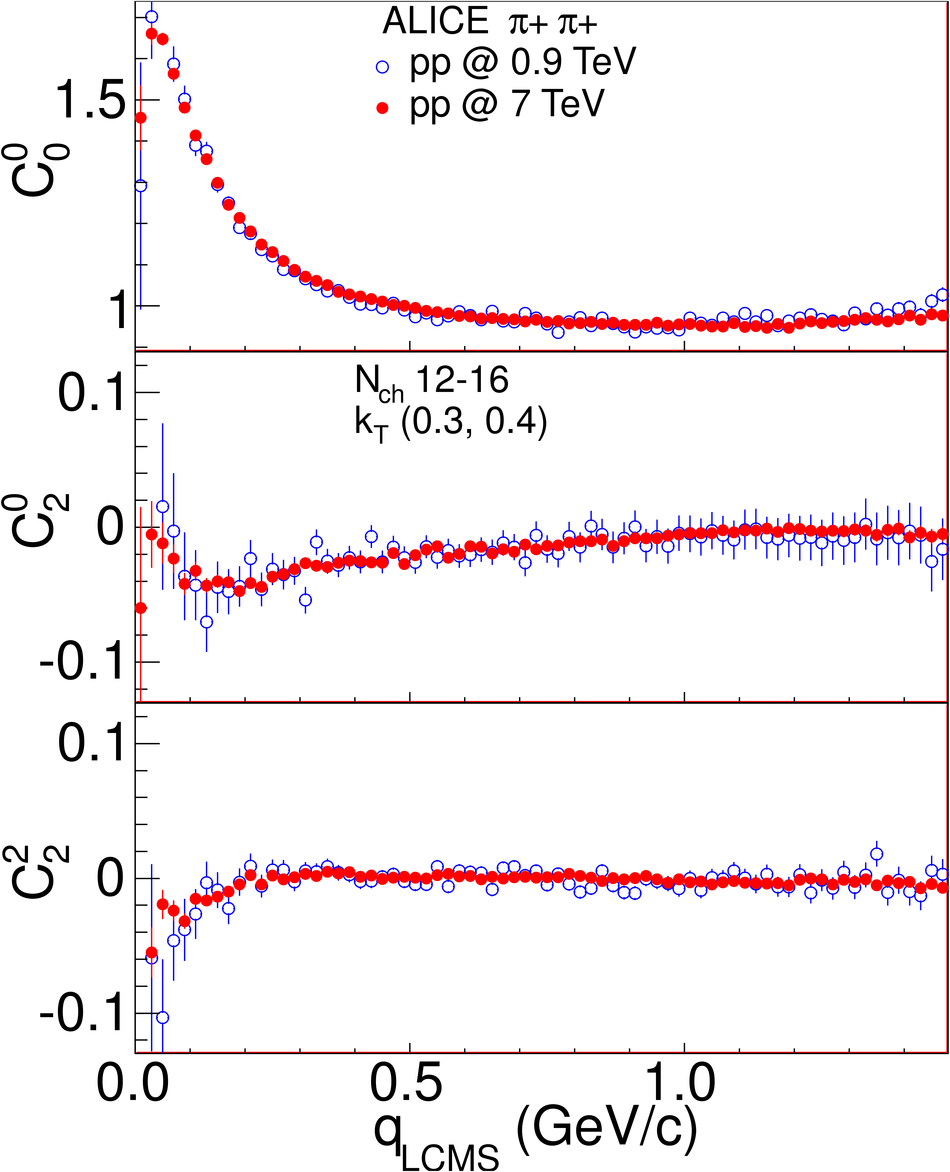## Figure 6

 Moments of the SH decomposition of the correlation functions for events with $12 \leq N_{ch} \leq 16$, pairs with $0.3 < k_{\rm T} < 0.4~$GeV/$c$. Open symbols are PYTHIA MC simulations (Perugia-0 tune), closed symbols are ALICE data from $\sqrt{s} = 7~$TeV collisions## Figure 7

 Summary of the MC simulations for selected multiplicity and $k_{\rm T}$ intervals, open symbols are a simulation at $\sqrt{s} = 7~$TeV, closed symbols at $\sqrt{s} = 0.9~$TeV. Dashed lines are Gaussian fit to the simulations to determine the background parameters (see text for details)## Figure 8

 Comparison of the correlation functions for $\pi^{+}\pi^{-}$ pairs at $\sqrt{s} = 7~$TeV (closed symbols) to the PYTHIA MC simulations (open symbols), in selected multiplicity and $k_{\rm T}$ intervals. The plot is made as a function of $q_{inv}$ instead of $q_{LCMS}$ so that the resonance peaks are better visible## Figure 9

 Moments of the SH decomposition of the correlation functions for events with $23\leq N_{ch} \leq29$ and pairs with $0.3< k_{\rm T}< 0.4~$GeV/$c$. The dashed line shows the Gaussian fit, the dash-dotted line shows the background component, the dotted line show the femtoscopic component.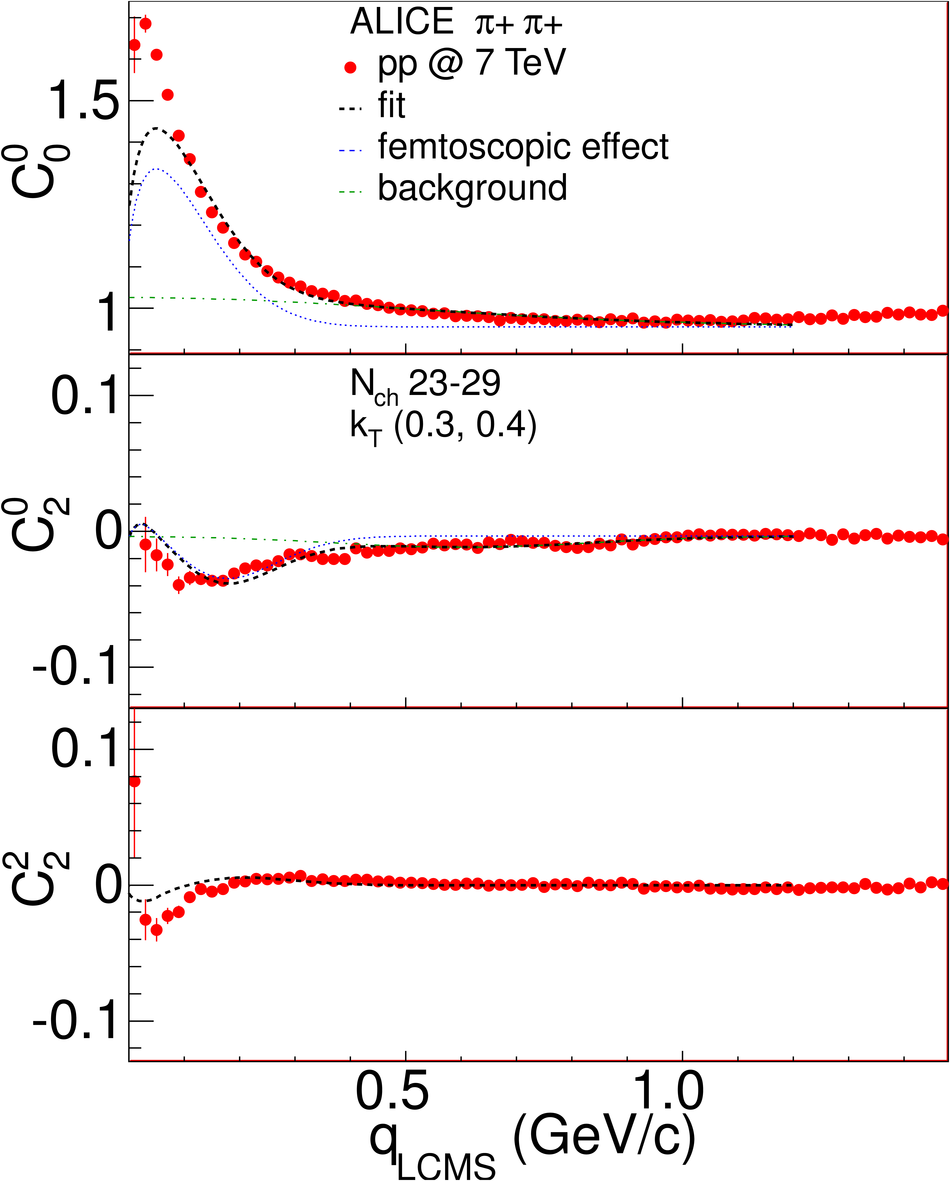## Figure 10

 Projections of the 3D Cartesian representations of the correlation functions for events with $23\leq N_{ch}\leq29$ and pairs with $0.3< k_{\rm T}< 0.4~$GeV/$c$. To project onto one $q$-component, the others are integrated over the range $0-0.16~$GeV/$c$. Dashed lines show analogous projections of the Gaussian fit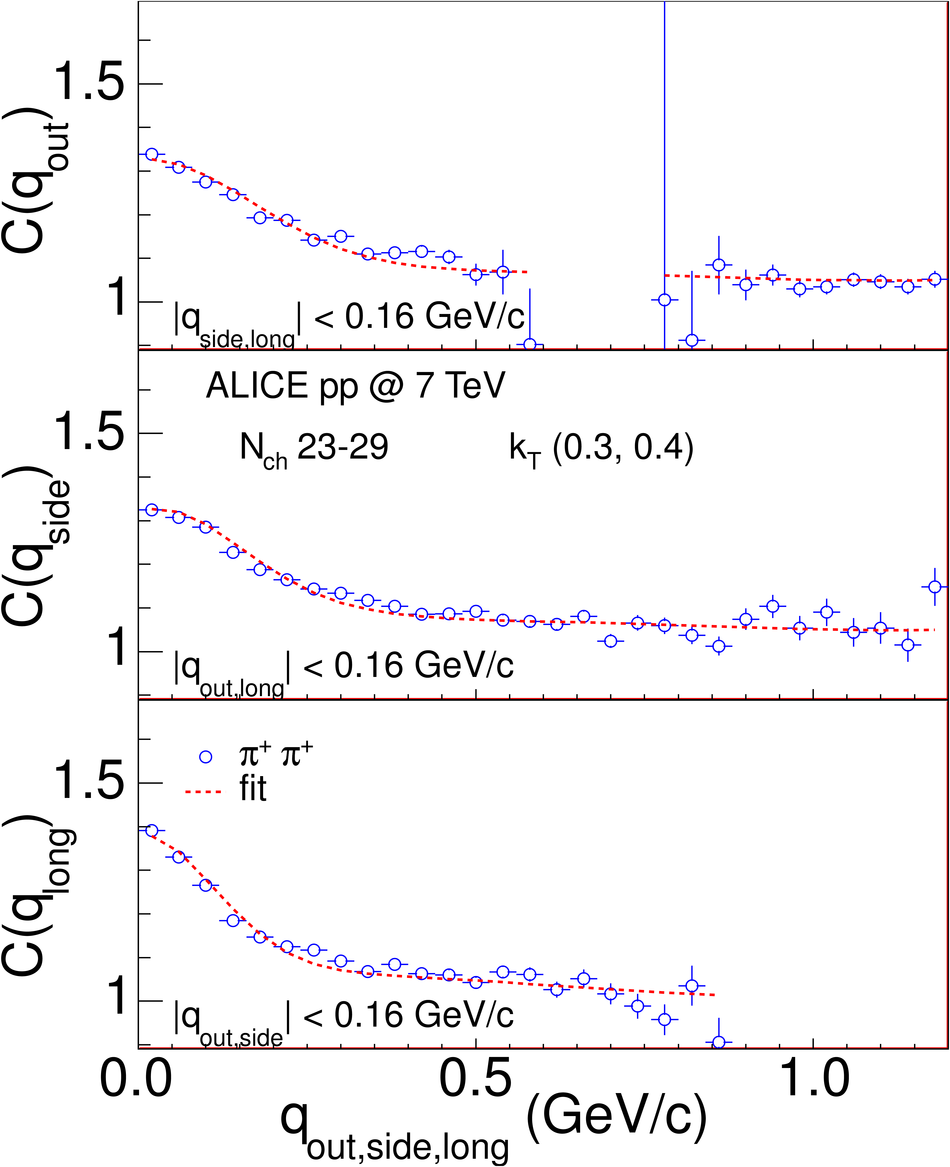## Figure 11

 Parameters of the 3D Gaussian fits to the complete set of the correlation functions in 8 ranges in multiplicity and 6 in $k_{\rm T}$ for \pp collisions at $\sqrt{s} = 7~$TeV, and 4 ranges in multiplicity and 6 in $k_{\rm T}$ for \pp collisions at $\sqrt{s} =0.9~$TeV. All points at given $k_{\rm T}$ bin should be at the same value of $k_{T}$, but we shifted them to improve visibility. Open black squares show values for pp collisions at $\sqrt{s} = 200~$GeV from STAR . Lines connecting the points for lowest and highest multiplicity range were added to highlight the trends## Figure 12

 Gaussian radii vs event multiplicity, for $\sqrt{s} = 0.9~$TeV and $7~$TeV. Panel a) shows $R^G_{out}$, b) shows $R^G_{side}$, c) shows$R^G_{long}$ and d) shows $R^G_{out}/R^G_{side}$ ratio. Lines show linear fits to combined $\sqrt{s}=0.9~$TeV and $\sqrt{s}=7~$TeV points in each $k_{\rm T}$ range## Figure 13

 Gaussian radii as a function of $\left< {\rm d}N_{ch}/{\rm d}\eta\right>^{(1/3)}$, for $\sqrt{s} = 0.9~$TeV and $7~$TeV,compared to the results from (heavy-)ion collisions at RHIC [32,33] and SPS . Panel a) shows $R^G_{out}$, b) shows$R^G_{side}$, c) shows $R^G_{long}$. All results are for $\left< k_{\rm T}\right>=0.4~$GeV/$c$, except the values from thePHENIX experiment, which are at $\left< k_{\rm T}\right>=0.45~$GeV/$c$## Figure 14

 Exponential-Gaussian-exponential fit example for events with $23 \leq N_{ch} \leq 29$, pairs with $0.3 < k_{\rm T} < 0.4~$GeV/$c$. SH representation. Dotted line shows the femtoscopic component, dash-dotted shows the background, the dashed line shows the full fit.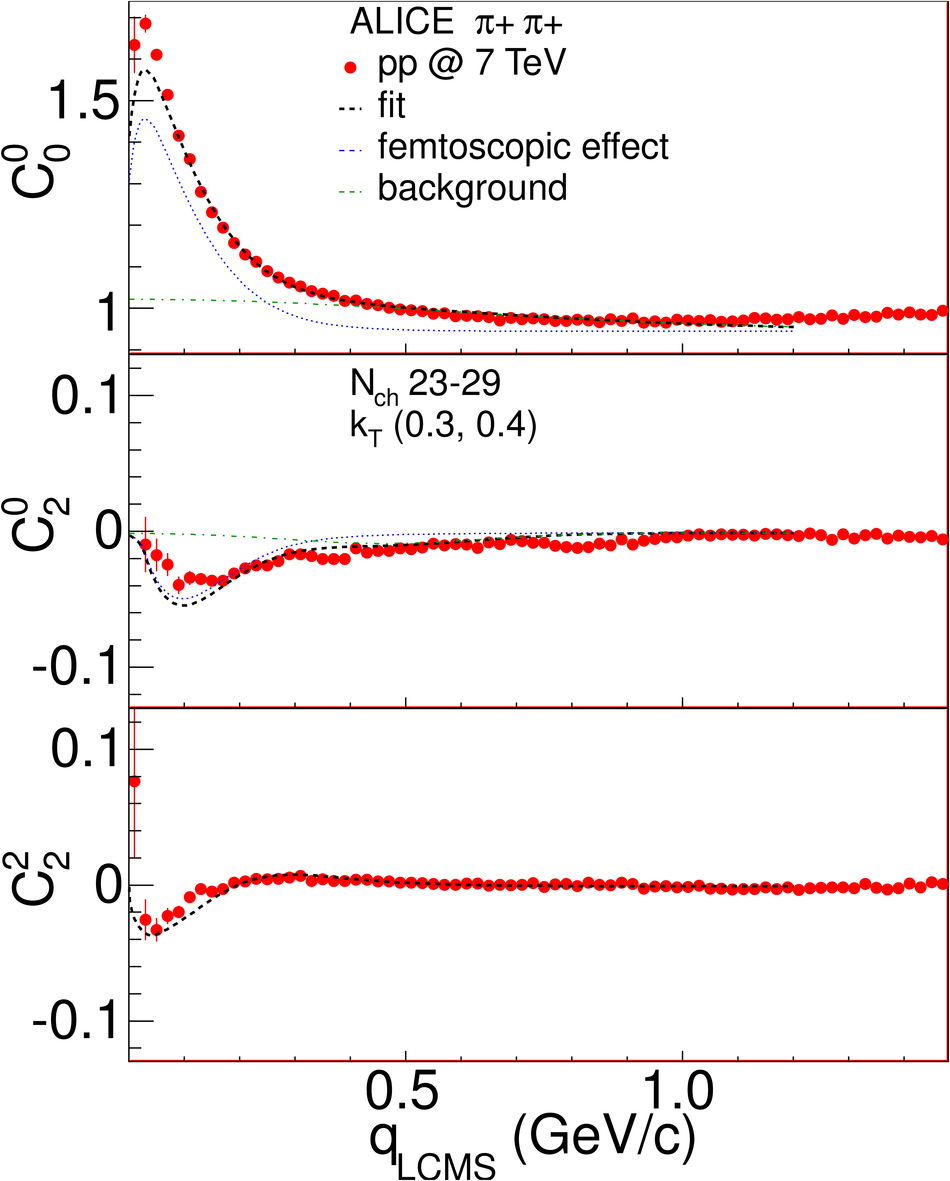## Figure 15

 Exponential-Gaussian-exponential fit example for events with $23 \leq N_{ch} \leq 29$, pairs with $0.3 < k_{\rm T} < 0.4~$GeV/$c$. 1-dimensional projections of the Cartesian representation are shown, the other $q$ components were integrated in the range $0-0.16~$GeV/$c$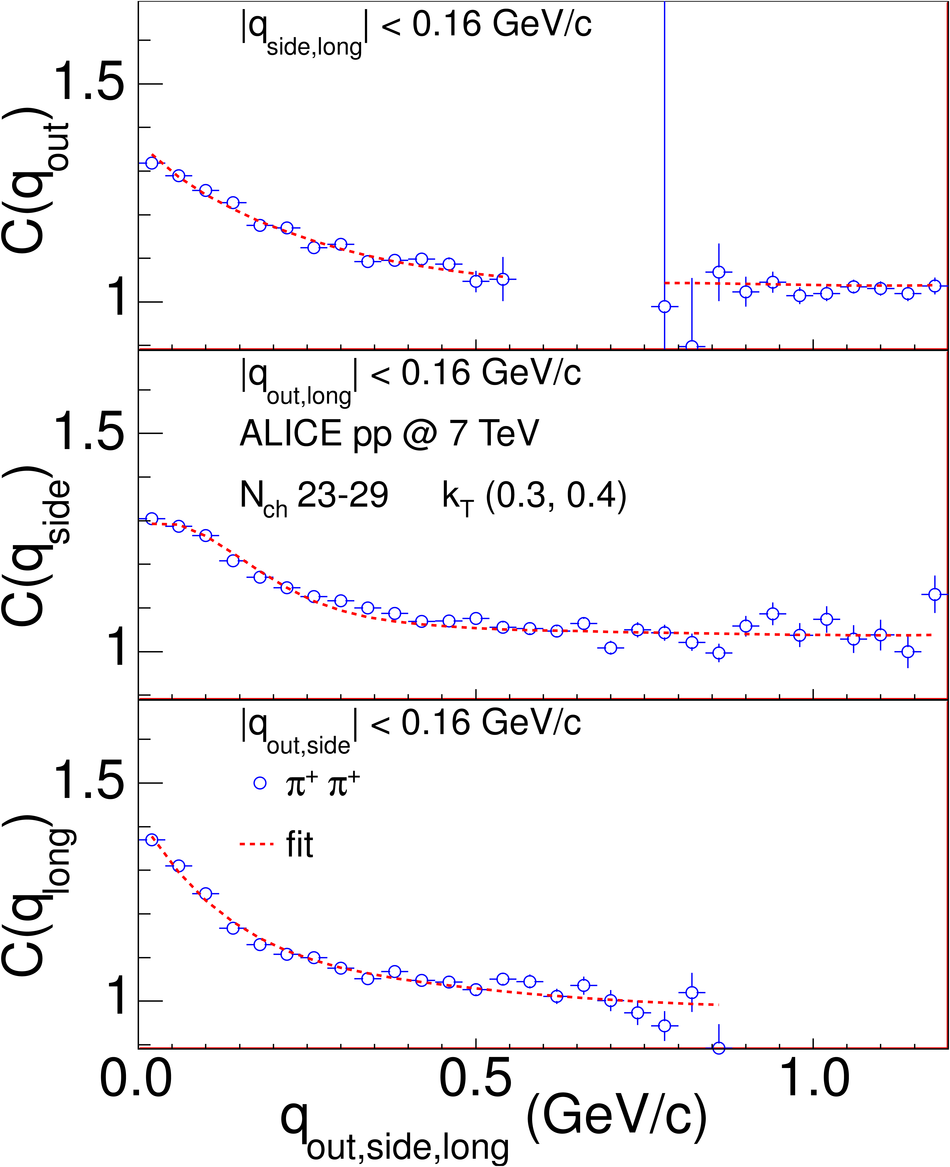## Figure 16

 Non-gaussian fit radii (see Eq.~\eqref{eq:Cege}) as a function of pair momentum $k_{\rm T}$ for all multiplicity ranges and for two collision energies. Panel a) shows $R^{E}_{out}$, panel b) shows $R^G_{side}$, panel c) shows $R^E_{long}$, panel d) shows $R^E_{out}/R^G_{side}$ ratio. All points at given $k_{\rm T}$ bin should be at the same value of $k_{T}$, but we shifted them to improve visibility## Figure 17

 1-dimensional $R_{inv}$ radius for all multiplicity and $k_{\rm T}$ ranges for the $\sqrt{s} = 0.9~$TeV data. The points for different multiplicities were slightly shifted in \kt for clarity. The systematic error, typically on the order of 10% is not shown. Closed and open stars show the previously published result from for two ranges of the multiplicity $M$## Figure 18

 1-dimensional $R_{inv}$ radius versus multiplicity and $k_{\rm T}$ for the$\sqrt{s} = 7~$TeV data. The points for different multiplicities were slightly shifted in \kt for clarity. The systematic error, typically on the order of 10% is not shown .